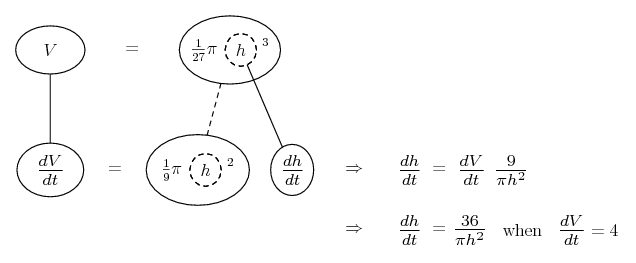# Thread: Word Problem

1. ## Word Problem

A tank in the shape of a right circular cone is being filled with water at a rate of 5 ft^3 per minute, but water is also flowing out at a rate of 1 ft^3 per minute. The tank is 60 feet deep and 40 feet across the top.

A) How quickly is the depth of the water changing when the water is 42 ft deep?

B) If the tank is empty, how long will it take for the tank to be 1/2 full?

C) How deep is the water when the tank is 1/2 full? How quickly is the depth of the water changing when the tank is 1/2 full?

Im really not sure if these are right

A) dv/dt=5-1=4, h=60, r=20
find dh/dt when h=42
h/r=60/20, r=h/3, dr/dt=1dh/3dt
60/20=42/r r=14ft.

V=pr^2h/3 dv/dt=p/3[2rhdr/dt+r^2dh/dt]
4=p/3[2(14)(42)(1dh/3dt)+196dh/dt]
4=p/3[392dh/dt+196dh/dt] = p/3[588dh/dt] = dh/dt=1/49p ft/min.

B) V=pr^2h/3 p(20)^2(60)/3 = 8000p(1/2) = 4000p
dv/dt=4ft/min if 1 min for 4ft, 16 hours 40 min for 4000ft

C) find h when V=4000
3v/pr^2=h h=3(4000)/p(20)^2 = 12000/400p = 30pft^3

find dh/dt when h=30
4=p/3[2(20)(30)(1dh/3dt)+20^2dh/dt]
4=p/3[800dh/dt]
dh/dt=1/66.67p ft/min.

2. A and B look correct.

For C, using the formula

$\displaystyle V=\frac{1}{3}\pi\left(20\cdot\frac{h}{60}\right)^2 h=\frac{1}{27}\pi h^3,\;\;\;\;\;\mbox{(Corrected)}$

I derived

\displaystyle \begin{aligned} \frac{1}{27}\pi h^3&=4000\\ h^3&=\frac{4000\cdot 27}{\pi}\\ h&=30\sqrt{\frac{4}{\pi}}. \end{aligned}

3.Originally Posted by Scott HA and B look correct.

For C, using the formula

$\displaystyle V=\frac{1}{3}\pi\left(20\cdot\frac{h}{60}\right)^2 h=\frac{1}{27}\pi r^3,$

I derived

\displaystyle \begin{aligned} \frac{1}{27}\pi h^3&=4000\\ h^3&=\frac{4000\cdot 27}{\pi}\\ h&=30\sqrt{\frac{4}{\pi}}. \end{aligned}
wow thanks! I didn't know about that formula. Is there one I could use for the second part of C? My answer for that looks completely wrong.

4. I corrected $\displaystyle \frac{1}{27}\pi r^3$ to $\displaystyle \frac{1}{27}\pi h^3$.

To find the rate of change of the depth at $\displaystyle h=30\sqrt{\frac{4}{\pi}}$, we may use the Chain Rule:

$\displaystyle \frac{dV}{dt}=\frac{d}{dt}\left(\frac{1}{27}\pi h^3\right)=\frac{1}{9}\pi h^2\frac{dh}{dt}.$

5. It's the same cone-volume formula that you used successfully in part A, but Scott has made things easier by eliminating r because it's just h/3. You could have done the same in part A (you pointed out the ratio) but you used the product rule instead. In part C you need the neater version, which Scott has given you as

$\displaystyle V = \frac{1}{27} \pi h^3$

which is of course correct, but beware his typo where he states it with r in place of h.

Now differentiate V with respect to h, and use the chain rule to deduce dh/dt. You need to restore the pi which you dropped from your value for the half-full volume during part B, and consequently you also want to remove pi from Scott's corresponding value of h.

Just in case a picture helps organise the chain rule...

Spoiler:... where... is the chain rule. Straight continuous lines differentiate downwards (integrate up) with respect to x, and the straight dashed line similarly but with respect to the dashed balloon expression (the inner function of the composite which is subject to the chain rule).

Edit: sorry Scott, thought you weren't online ... however, perfectstorm does want to note the rogue pi.

__________________________________________

Don't integrate - balloontegrate!

Balloon Calculus: Gallery

Balloon Calculus Drawing with LaTeX and Asymptote!

6. I think I got it now thanks a lot Scott, and Tom your picture helped!

#### Search Tags

problem, word